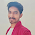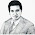## Index

 STA & SI Chapter1 Chapter2 Chapter3 Chapter4 Chapter5 Chapter6 Chapter7 Chapter8 Introduction Static Timing Analysis Clock Advance STA Signal Integrity EDA Tools Timing Models Other Topics

 Extraction & DFM Chapter1 Chapter2 Chapter3 Chapter4 Chapter5 Chapter6 Introduction Parasitic Interconnect Corner (RC Corner) Manufacturing Effects and Their Modeling Dielectric Layer Process Variation Other Topic

## Monday, February 20, 2012

### Design constraint : Maximum Fanout

 7.4a 7.4b 7.4c Maximum Transition Time Maximum Fanout Maximum And Minimum Capacitance

Design Constraints are divided into several parts because it’s really a wide and important topic. I want to discuss this in detail. I have also noticed that lot of information is present in internet but those are bits and pieces. So I am trying my best to cover everything here in a proper way. Let me know in case any of you have any feedback.

• Part 1a ->  Basics of Design Constraints and Details of "Maximum Transition Time" (max_transition)
• Part 1b -> Maximum Fanout Constraint. (max_fanout)
• Part 1c ->  Maximum (and minimum) capacitance (max_capacitance and min_capacitance)
Note: Rest Of the parts is still in development.

In the last blog we have discuessed that Design Constraints are of 2 types:
• Design Rule Constraints
• Optimization Constraints
And further Classification of Design Rule Constraints are -- 4 types:
• Maximum transition time
• Maximum fanout.
• Maximum (and minimum) capacitance.
Details of Maximum Transition Time - we have discuessed in last blog. In this part we will discuss about the "Maximum Fanout".

Maximum fanout:

• The maximum fan-out of an output measures its load-driving capability:
• It is the greatest number of inputs of gates to which the output can be safely connected.
• Fanout load is a dimensionless number
• This info is present in the .lib file. Please see the below snapshot of .lib with respect to one cell definition.
• “max_fanout” is available only for “output” pins.
• Whether the circuit is violated or not – calculated as per the following e.g
• Way to calculate:
• Sum up all the fanout loads for inputs driven by a pin (driving pin).
• If the sum of the fanout loads is not more than the max_fanout value, the net driven by X is valid or say not violated , else you have to make changes in your design by adding a buffer or anyother way.

As in the above figure : the max_fanout of X defined aas per the l.lib file is 20. Now if few loads are connected as the output at X then first calculate total fan loads.

So Total Fanout Load is  1.0 +1.0 +3.0 +2.0 = 7.0
Since max_fanout of X > 7.0 - so no violation.

Now let assume that you have set the fanload of OUT1 18.0 ( by using set_fanout_load 18.0)
then Total Fanout Load is 1.0+1.0+18.0+2.0 = 22.0
Since max_fanout of X < 22.0 - so its violation.

Similarly It may be that in you reset the max_fanout of X to 6.0 (by using set_max_fanout 6.0 )
then Toatal Fanout Load is 1.0 +1.0 +3.0 +2.0 = 7.0
Since max_fanout of X < 7.0  - so its violation.

Now same question : What's the need/importance/significance of this parameter (max_fanout) in the design?

Typically manufacturer defines the maximum input current for a particular cell at each logic level. So fanout of a cell A is the max no of input that can be connected to an output (of A) before the current requirement by any of the input exceeds the current that can be delivered by the output while maintain the correct logic level.  ( very big sentance  :)  Read it 4-5 times.. :) ). Actually these are very basics- But its very important to understand this here. :) .. (So I have copied this from Ref1.)

The fan-out depends on the amount of electric current a gate can source or sink while driving other gates.
• When an output pin is HIGH, the IIH requirements for all receivers must add-up  to be ≤ to the driver's IOH
• When an output pin is LOW, the IIL requirements for all receivers must add-up  to be ≤ to the driver's IOL.

Given that an output of any logic device can go either HIGH or LOW (High-impedance is not relevant), and hence exhibit either IOH or IOL, respectively, the Fan-Out is the minimum of two ratios:
Fan-Out = min ( IOH/IIH, IOL/IIL )

For example, Input and output currents are the following. Recall that negative current values indicate current flowing out of the gate while positive current values indicate current flowing into the gate:
• IOH = -400 µA  (i.e., output can source a maximum of 400µA)
• IOL = 16 µA (i.e., output can sink a maximum of 16µA)
• IIH = 40 µA (i.e., input can sink a maximum of 40µA)
• IIL = -1.6 µA (i.e., input can source a maximum of 1.6µA)
Therefore the fan-out is min ( 400/40, 16/1.6) = min (10, 10) = 10. In other words, each gate can drive 10 other gates of same type without getting out of its guaranteed range of operation. If more than 10 gates were connected, the output voltage levels will degrade and the gate will slow down.

What if there were different IC families, where the output was coming from one family while the other inputs related to different logic families? In this case, add IIH for all inputs connected to an output. The sum must be less than the output's IOH. Then add IIL for all inputs connected to an output. The sum must be less than the output's IOL.
The following diagram illustrates the principle of Fan-Out for both HIGH and LOW outputs:

When the NOR gate output is HIGH, the output bin behaves as a current source since IOH flows out of the driver gate and into the set of driven gates. The current IOH equals the sum of all input currents indicated by IIH, flowing into the driven gates. In other words, IOH = ∑IIH.

When the NOR gate output is LOW, the output bin behaves as a current sink since IOL flows into the gate and out of the driven gates. The current IOL equals the sum of all input currents indicated by IIL, flowing out of the driven gates. In other words, IOL = ∑IIL.
For example, IOL (MAX)  = 16mA and IIL (MAX) = -1.6mA (negative current values indicate current flowing out of the gate). Therefore, fan-out is 16/1.6=10:

Snapshot of .Lib File (Liberty File)

cell (<cellname>) {
cell_leakage_power : 3.748077e-03;
threshold_voltage_group : "si38p" ;
area : "8.775" ;
….
abc_cell () {

pin (Z) {
direction : "output";
}
pin (CP) {
direction : "input";
}
pin (D) {
direction : "input";
}
….
}
pin (Z) {
direction : "output";
related_bias_pin : "VDDB VSSB";
max_capacitance : 0.334971 ;
max_fanout : 20 ;

timing () {
index_1 ("1.0, 1.5, 2.0") ;
values ("1.0, 1.5, 2.0") ;
}
....
}
}

In the next blog (part) we will discuss rest of the Design rule Constraints.

1.Thanks a lot for sharing this kind of knowledge sir, excellent . I had clear a lot of doubts from this information.

2." maximum input current for a particular cell at each logic level."

this should be either minimum input current or maximum output current.

1.No Dear .. even max input current is also required

3.4.sometimes I found many designers limit the primary inputs fan out to be 1

set_max_fanout 1 [all_inputs]

but i can not understand why. Kindly can you explain it.

Thanks

5.Hi Sir,
Thank's a-lot for sharing the information & the knowledge that you have which is best of its quality.I would like to thank you once again for taking time in solving queries by giving reply..which rarely people do....I only wish that your knowledge will helps other's in achieving their goals.... and you may live prosperously... by adding more & more posts...

Thanks & Regards

6.what is the diffence between max_fanout and max_cap

1.fanout relates to current whereas cap relates to strength

7.What concept using in this method

8.I have some confusion. While I understand that max_fanout attribute is associated with output pin of a cell and fanout_load attribute is associated with input pin(s) of a cell, what I find strange and counter-intuitive is as follows:

The command set_max_fanout which sets attribute max_fanout is executed for input ports instead of output ports. Similarly the command set_fanout_load which sets attribute fanout_load is executed for output ports instead of input ports.

I can confirm this because this is what is mentioned in Synopsys Design Compiler user guide. Can you explain whats going on here and clear this confusion for me ?

Thanks

1.I am not the author but please allow me to attempt answering your question. I think the key here is "port". In Synopsys tools input "port" means a driver, while input "pin" means a load, similarly for output ports/pins.

What this entails is that when you set_max_fanout you are telling the tool what to expect of the other module that is going to drive this input port of current top module, and when you set_fanout_load you are telling it what to expect of the module that is driven by current top module's output ports.

Hopefully this helps.

9.Hi.. I had a quick question. My aim is to use a splitter circuit (qin, qout1, qout2 ) such that qout1=qout2 = qin. So basically I want the fanout of all gates in the library in my liberty file to be only 1. Following your text, I set max_fanout to 1 for all output pins in the circuit. However, when I convert from behavioral to structural verilog of a schematic using this liberty file, the splitter is inserted only for flip flops. For normal gates like and, nand, it's ignoring the splitter insertion. Could you please advise . Thank you

10.In the diagram which you explained for max fanout, the nand gate input won't take any current from nor gate output pin right. Because it is going to the gate terminal of NMOS AND PMOS. Then how we can conclude based on current?